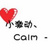# 怎么构造Face_range？

Stroman 发布于 03/10 16:15

0#include <CGAL/Simple_cartesian.h>
#include <CGAL/Polyhedron_3.h>
typedef CGAL::Simple_cartesian<double> Kernel;
typedef CGAL::Polyhedron_3<Kernel> Polyhedron;
int main() {
// 构造Polyhedron对象
Polyhedron P;
// 添加顶点
P.make_tetrahedron();
// 构造Face_range对象
auto faces = P.faces();
// 遍历Face_range
for (auto f = faces.begin(); f != faces.end(); ++f) {
// 处理Face_handle
}
return 0;
}

#include <CGAL/Iterator_range.h>
#include <CGAL/iterator.h>
typedef CGAL::Simple_cartesian<double> Kernel;
typedef CGAL::Polyhedron_3<Kernel> Polyhedron;
int main() {
// 构造Polyhedron对象
Polyhedron P;
// 添加顶点
P.make_tetrahedron();
// 手动构造Face_range对象
auto faces = CGAL::make_iterator_range(CGAL::counting_iterator<Polyhedron::Face_index>(0),
CGAL::counting_iterator<Polyhedron::Face_index>(P.size_of_facets()));
// 遍历Face_range
for (auto f = faces.begin(); f != faces.end(); ++f) {
// 处理Face_handle
}
return 0;
}